INVESTIGATING STATISTICAL CONCEPTS, APPLICATIONS, AND METHODS, Second Edition

NOTES FOR INSTRUCTORS

February, 2015

Chapter 1        Chapter 2        Chapter 3        Chapter 4

CHAPTER 3

This chapter focuses on quantitative variables. We begin with explorations of distributions with quantitative variables and there are investigations that explore the sampling distribution of a sample mean and introduce the t-distribution and one-sample t-tests as well.  You have some options in how much detail you want to discuss about the t-distribution.  Then the chapter moves into comparing groups.  Following the structure of the previous chapter, we first discuss comparing two population parameters (modelling random sampling, Section 2) but then contrast that with comparing two treatment parameters (modelling random assignment, Section 3). You will need to make some choices as to how many of these simulations you want students to carry out themselves vs. you demonstrating vs. you talking them through the concept. Section 4 looks at matched pair designs, through simulation and then through the paired t test.  (By this point in the course, students can be asked to consider advantages and disadvantages of different analysis approaches. There is also an optional example on the sign test.) Investigation 3.13 also looks at paired categorical data through McNemar’s test.

There is an optional on-line investigation exploring the basics of bootstrapping (pdf) and some follow-up homework exercises.  At the end of the HW are exercises on normal probability plots.

Section 1: Quantitative data

In earlier versions of the text we focused solely on comparing groups and attempting to get at issues of distribution and variability within that context alone. We start with a small initial investigation that focus on one quantitative variable descriptively. If your students are quite familiar with descriptive statistics, you may choose to go through this material very quickly.  The second investigation focuses on the sampling distribution for a sample mean.  This can be used to build intuition only or you can continue to Investigation 3.3 to illustrate one-sample t-procedures and prediction intervals. The applet Exploration can be used instead of using R or Minitab to illustrate repeated confidence intervals.

Investigation 3.1: How Faithful is Old Faithful?

Timing: This can be one 50-min class period, depending on how smoothly the technology goes, but giving them lots of time to create and explore the graphs and descriptive statistics on their own.  Can also be assigned for an out of class exercise. If you are using R, you will need a bit more lead time on importing and attaching data. (Working with missing values may come up down the road as well. One approach is to use as.numeric(as.character(x)) and then na.omit(x) but see Investigation 3.12 notes.)

Materials needed: OldFaithful dataset (stacked) and method for students to import into technology. We also encourage you to show the Old Faithful webcam.

We find this data set to be interesting to students and rich enough to focus on many different aspects. Previously we have focused on introducing measures of spread with this context, but the distributions also allow interesting discussions of shape and center as well, and what each of these can tell us about the research question.

You will probably need to spend some time at the start reviewing how you want students to access quantitative data files.  The text assumes reading from the clipboard but downloading files is probably a better way to go if your institution has good file server access. Most of this investigation is learning how to use the technology, but students can still tie their observations directly to the context and comparing the distributions. If your students have no past experience with descriptive statistics, consider spending more time on measures of spread.  Practice Problem 3.1B is especially good for predict-and-test type class discussion. (Students often confuse variability with bumpiness or variety.)

You may also want to consider having students work with probability plots in a homework assignment.  We also caution students not to use the term “even” when they mean symmetric as statisticians will hear “uniform.”

Technology notes: R can be a pain if you want to load in a dataset with missing values. You will also need to use a package for histograms (the text assumes RStudio, with only R you will need to load the package first). You will probably also need to mention at some point that different statistical packages calculate quartiles/box hinges in different ways and won’t always match each other or by hand calculations. There is now an “iscamboxplot” function that will use the quartiles from the iscamsummary output (using quartiles) in creating the boxplots, rather than R’s default of hinges (which better match “by hand” calculations of quartiles). Note that with iscamdotplot and iscamboxplot you can also enter variable names, e.g., iscamdotplot(time, year, names=c("intereruption times", "year"))

The Descriptive Statistics applet has more features now as well, including changing bin widths of histograms and overlaying boxplots.  After you check “stacked” you need to use the button to specify whether the categorical grouping variable (explanatory variable) is the first column or the second column.

Investigation 3.2: The Ethan Allen

Timing: This can also be about a 50-min class period.  If you need to supplement, you can also talk about variability in means vs. individual observations (e.g., Why do we diversify a stock portfolio? Why do some sporting contests (e.g., rodeo) average over scores rather than just having one score?).

Technology: Sampling from a Finite Population applet (javascript).

A true story of a tour boat that sank.  There were no deaths and you can find pictures of the incident online.  We designed this investigation to continue having students focus on expected characteristics of distributions and to emphasize the distinctions between sample, population, and sampling distributions.  The change from total weight to sample weight is not required, but allows us to focus only on the distribution of sample means.  The applet asks you to paste in different hypothetical populations. You can replace or extend the sampling from the applets to having them generate random samples from different probability models using R or Minitab. You may also want to supplement the statement of the Central Limit Theorem with derivations of the formulas for the mean and standard deviation of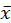(example).  The key will be having students fill in the table in (r) and verifying the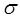/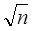formula vs. the applet results. You might also want to ask students to investigate for each of the population how small the sample size can be before the sampling distribution would not be considered approximately normal.  Also be warned it will take students a while to get out of the habit of using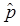and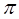. In (w), you may prefer to overlay the normal distribution rather than going to the Normal Probability applet.

Investigation 3.3: Healthy Body Temperatures (Modified)

Timing: We split this into two investigations. The first investigation may take just 30 minutes, but may lead to additional discussion.

This investigation was revised (Fall 2014) to focus a bit more on use of the t distribution as a mathematical model for the standarized statistc (using the sample standard deviation in place of the population standard deviation). Students see that the normal distribution underestimates tail probabilities, especially for small sample sizes. Showing the coverate rate of t-intervals may be even more convincing. (This part of the investigation helps students see why the t critical value is necessary while reviewing the frequentist interpretation of confidence level.) You have some flexibility in how much detail you want to cover here. The t distribution and similar issues are returned to when discussing the difference in sample means.

You may want to answer question (a) from Investigation 3.4 after defining the symbols.

Investigation 3.4: Healthy Body Temperatures (cont.) (Modified)

Timing: The first part of the investigation applies the results in the previous section (perhaps 30 minutes) but then also discusses and contrasts prediction intervals.

This investigation provides a straight forward application of the CLT with a genuine research question about whether 98.6 is the “right” temperature to focus on. Students first complete the calculations by hand and then verify using technology. The end of the investigation focuses on the distinction between confidence intervals and prediction intervals.  Students will need to know how to find t critical values to calculate the prediction intervals by hand.

Section 2: Comparing Two Population Means

The following investigations have been reordered to better distinguish between taking independent random samples and using random assignment.

Investigation 3.5: Left-handedness and Life Expectancy

Timing:  The timing of this investigation can be quite variable depending on how much you want them carrying out the simulations themselves.

Students will probably find this context interesting and it is worth spending some time talking about the data collection issues and the implications.  In this case, it is more natural to consider the data as arising from random sampling rather than random assignment. They use simulation to explore the test statistic and consider both a normal and a t distribution as a model, and how these models change with sample size.  The formula for the standard deviation of the difference in sample means is not derived but instead results are compared to the simulation results. It might be worth stepping them through the logic of combining the standard deviations before showing them the formula.   Once you feel they understand the t-distribution, you can move to using R or Minitab to perform two-sample t-tests.  One issue to focus on here is stacked vs. unstacked data.

If you assign this practice problem, you will probably want to give them more specific instructions. Part (a) hints at the technical conditions of the t-test and in (b) it may be interesting to discuss with student the benefits of equal sample sizes but with the reminder that because we are working with means (and/or proportions) this is not a requirement of a good study design.

Investigation 3.6: Left-handers and Life Expectancy (cont.)

Timing: Can be done in about 15-min or you can use the last questions as a jumping off point for further discussion.

This investigation uses the data from the previous investigation to focus on the analysis while exploring the effects of different factors on the statistical significance of the results.  Students may bring up the “catch” before you do. The purpose of this investigation is to explore the effects of sample size and within group variability on the p-value (this is a good example of the common habit of many studies to report means but not sample standard deviations).  Make sure students take advantage of the technology to complete these calculations fairly quickly so they can focus on the change in p-value.  Students should do fairly well in (d) but we’ll see if they remember how to interpret standard deviation in terms of the empirical rule (though lifespans are probably not symmetrically distribution). Questions (g)-(k) serve as a good review/reminder of Types of Error.

Section 3: Comparing Two Treatment Means

Investigation 3.7: Lingering Effects of Sleep Deprivation

Timing: 45 minutes (though can probably do 3.7 and 3.8 together in one day)

Materials needed: 21 index cards, Randomization Test (aka Comparing Groups on a Quantitative Reponse) applet

Now we move into comparing two groups arising from random assignment rather than random sampling. At this point, students may be able to walk you through how to design a simulation to explore the issue. You can focus on describing a tactile simulation or writing out “pseudo-code.”  We think it is still worth the time to do a tactile simulation at this point. (Make sure everyone subtracts in the same way, deprived – unrestricted.) You can emphasize that it won’t be as easy to count all the “tables more extreme” as we need to consider the average outcome in both groups this time (well, at least one) and you need to know the numerical values, not just the number of yeses and no’s this time. The applet has some nice visuals. It also allows you to easily change to the difference in medians but we now put off that discussion to the end of the chapter though you could preview it to show the flexibility of the randomization approach. You may also want to follow up the applet simulation with technology instructions for doing the simulated randomization distribution in R or Minitab.  The text also discusses the “exact” probability distribution and you can emphasize how cumbersome and really unnecessary it is to obtain that distribution. The simulation gives pretty close results and works for any statistic they choose to use.  In (h), you may want caution them when using the standard form (in terms of population parameters) of the null and alternative hypotheses with an experimental study design. (We no longer define a distinct symbol for a treatment effect.)

Technology notes: If you plan to have them use R or Minitab for these simulations later (may not be necessary on top of the Randomization Test applet), be sure to have them save these scripts/txt files. In R, though the code presented in the text may be efficient, it might make more sense to the students to randomly mix the response variable values. You can also add the visual of showing the trial by trial boxplots and/or dotplots with each reshuffling.

Investigation 3.8: Lingering Effects of Sleep Deprivation (cont.)

Timing: 50 minutes (though can be done much quicker)

Materials: You will probably want output of the Randomization Test available to show and discuss with students. This link defaults to the Sleep Deprivation data.

A continuation of the previous investigation, applying the normal model to get to the t procedures, which at this point in the course students may be expecting. (You may be able to stream line some of this discussion depending how much you talked about the t distribution earlier.) Again the formulas for the standard deviation are not derived but instead results are compared to the simulation results. This is a good time to remind students of the difference between number of samples (repetitions of the simulation) and sample size.  In particular, try to curb student assumption that large samples make the sample or population data more normally distributed.  You may not want to spend too long on the comparison to the t distribution as most students will not be as excited by the result as you are, but you can of course discuss the history with Gosset.  It might be interesting to note that the z distribution underestimates the p-value (and the standard deviation is an underestimate but that with the t distribution, it magically balances out just right).  We try to convince students of the merit of the t distribution by showing the p-value is closer to the exact p-value. (Students probably won’t come up with this way of deciding on their own.) You can discuss how the SE formula is not quite as good a model but still seems to be a reasonable approximation. Degrees of freedom will be pretty mysterious to students at this point. The text deliberately does not spend much time on degrees of freedom (the 17 is just given to them to use in (f)) but might be worth flashing them the formula and/or how min(n1, n2) – 1 is a conservative estimate (and what we mean by conservative and why that’s a reasonable way to err). You may also choose not to spend too much time on pooled vs. unpooled. The main idea you want to convey in this investigation is the reasons for now using the t distribution as the reference distribution. (You may want to contrast to how we analyzed the Ethan Allen study where we assumed we knew the population standard deviation in that case.)

Investigation 3.9: Ice Cream Serving Sizes

Timing: 30 minutes

This investigation begins as review but then also introduces the two-sample t-interval.  After this investigation might be a good time to again explore what is meant by “confidence level” and using simulations to explore the robustness of the t-intervals under different populations shapes (example applet here).

Investigation 3.10: Cloud Seeding

Timing: 60 minutes (You may want to collect the data for Investigation 3.10 during the preceding class period.)

Materials needed: Access to cloudseeding data file (stacked) and possibly Randomization Test applet.

This investigation explores a random experiment with a very skewed response variable which brings into question the appropriateness of the two-sample t-procedures.  Question (g) will probably be tough for them, but one approach is to consider using a statistic other than means, such as the more resistant median as a measure of center. You can draw the parallel with examining other statistics (like relative risk and odds ratio) at the end of Chapter 2. Students may also recall how we used a transformation to make a skewed distribution into a normal distribution in Chapter 2. You can then use R or Minitab or the Comparing Groups applet to make this modification. Students can easily calculate a p-value with the randomization test (remember to use the observed difference in group medians as the statistic), but what about a confidence interval? (Note, the Randomization Test applet linked to in the text does allow you to put in other values for the hypothesized difference in group means and will even illustrate the shifting in the distribution modeled by this nonzero treatment effect.)

Another approach is data transformation. As they saw before with a right skewed distribution the log transformation (rescaling) often works to “pull in” the larger values and create a more symmetric distribution. The last page also discusses back transforming the confidence interval into a ratio of population medians but you may not want to emphasize this detail with your students (though you can build on the multiplicative increase interpretation they used with relative risk and odds ratio). It is worth noting that the log transformation (essentially) preserves the median but not the mean.

The practice problem gives them a large population to sample from and compare the results of one sample to the actually known parameter values.  It also raises the issue of deleting outliers from a data set. May also want to emphasize how nice it is when the shape and spread are at least the same as the analysis just detects a shift in center.

Section 3: Matched Pairs Designs

The last section in this chapter focuses on paired data, both paired designs and analysis.  Again students have an option for exploring a randomization approach to estimate the p-value. A good theme to emphasize throughout this section is the role of variability on our analyses, and how the statistician’s goals are to explain or at least account for as much of this variability as possible.

Investigation 3.11: Chip Melting Times

Timing: May take two class periods, with the first focused on the paired design and descriptive statistics, and the second focused on statistical inference for paired data.

Materials needed: Semisweet and peanut butter chips, perhaps distributed through Dixie cups.  (Feel free to vary the types of chips, e.g., butterscotch.) Access to a timing mechanism. This is a fun data collection activity but to be honest probably won’t produce statistically significant results. You may want to have a back-up version of the data file. It is good for students to see insignificant results in general, but the advantages of pairing may not come out of this investigation by itself.

Before collecting the data, check for chocolate and peanut butter allergies as students will use both types of chips. It is worth giving very clear and consistent directions for how students are to carry out the study. You can also ask them to flip a coin or some other mechanism to decide which to melt first. (You may not even want to reveal yet that they will be melting both.)

You probably will not see a significant difference in the randomized comparative study design so that motivates exploring alternative study designs.  Try to elicit from students why it might be helpful to have each student melt both chips, beyond the increase in sample sizes.  When you collect the data, make sure you know which type of chip they melted first and the melting time for each chip separately.  The text assumes you end up with two columns of times and then tells students how to stack the data (in R) and then get the parallel dotplots.

For question (j), make sure they remember the “independent samples” condition of the two-sample t-procedure as well as not having utilized the information that the data are paired.  In question (k), we would hope to have a smaller standard deviation of the differences, but this is not always the case.  In (m), have them think about drawing lines to connect points where the lines all go in one direction vs. where the lines have lots of crisscrossing.

Investigation 3.12: Chip Melting Times (cont.)

Timing: 45 minutes

Materials needed: Matched Pairs Randomization applet

Now it’s time to estimate a p-value for the differences, based on a matched pairs design. You will again want to have some caution in defining the parameter.  Notice in (b) that a two-sided alternative is implied. See whether students can tell you how to design a simulation to mimic the randomness that was used in this study (the order of the chip), such as with a coin flip, assuming the melting times would have been the same no matter which chip they had.  They may also be able to set up “pseudo-code” for the randomization. The Matched Pairs Randomization applet show them a visual of the times swapping places within each row before plotting the new differences and the average difference. (Another good time to highlight the distinction between population, sample, and sampling distribution.) It also allows you to see the “lines pattern” in the paired data to compare the observed “slopes” to the simulated slopes.  The investigation then turns to the two-sample t-test and you can decide how much of the work you want the technology to do vs. having the students continue to focus on a one-sample t-test of the differences.

Investigation 3.13: Comparison Shopping

Timing: 30-40 minutes, this can expand if you choose to do more with data cleaning and exploration

Materials needed: Optional - Access to shopping data (may also want to consider making this a class data collection project)

In R, you will have to handle the missing values. This data set is small enough that they can be removed before importing into R.  You can also remove them once in R (e.g., edit(x) or fix(x)), but for the paired data, you will need to remove them from all three columns. You will probably also need to convert them to numerical values using as.numeric. The following can convert the * values to NaN that R will recognize.

·       For one set of values, the following will delete the missing values.  The output is the same without na.omit but the sample size will not reflect the number of missing values.

`na.omit(as.numeric(as.character(Luckys))))`

·       For paired data:

This induces NaNs in the columns.

combined=cbind(as.numeric(as.character(Luckys)),as.numeric(as.character(Scolaris)))

This deletes all of the rows that have a missing value for either store.

`newcombined=na.omit(combined)`

If you haven’t discussed different sampling plans yet, this is a good context.  What would be problematic about taking a simple random sample of items and then finding their prices, if you have to wonder around an unfamiliar store?  What would be an advantage to stratified sampling? Systematic sampling?

Once the data are cleaned (one of the few times we have a reason to remove an outlier – we can see that the items were not identical), the analysis is straight forward (though may also want to raise the issue about what to do when an item is on sale).  So then the investigation turns to the idea of a prediction interval. Emphasize to students that they have created an interval for the population mean, not for individual items. (At this point, many students will still have this misconception or that the confidence interval captures 95% of sample means.)  But they can create an interval for individual items if they take into account the additional item to item variability (in addition to the sample to sample variability).  Be sure to clarify to students how you would phrase an exam question in each case.

A follow-up to this investigation could look at a sign test on the positive/negative differences, which would be resistant to outliers in the data but would lose power by ignoring the magnitude of the differences. (See Example 3.4 and HW exercises.)

Investigation 3.14: Smoke Alarms

Timing: 30 minutes

Continuing the theme of pairing, this investigation does so again but with categorical data.  Students should be able to see the intuition behind using a binomial test on the number of pairs where the outcomes differed, providing a nice review of earlier material. You may want to trim some of the simulation details and focus on applying the binomial probability to find the p-value.

Again, remind students of the end-of-chapter materials.